# Kinetic Friction in a Block-and-Pulley System ③ 134 10 Consider the system shown in the figure...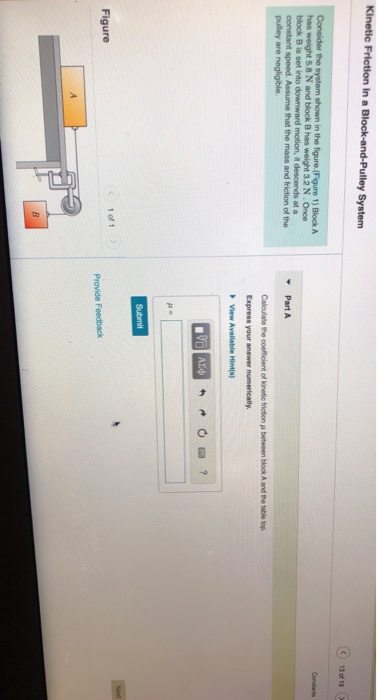Kinetic Friction in a Block-and-Pulley System ③ 134 10 Consider the system shown in the figure (Figure 1) Block A has weight 5.8 N and block Bhas weight 3.2 N . Once block B is set into downward motion, it descends at a constant speed. Assume that the mass and friction of the pulley are negligible Part A between block and the table top Calculate the coefficient of kinetic friction Express your answer numerically, View Available Hints) DVD AED 0 ? Submit Net Figure Provide Feedback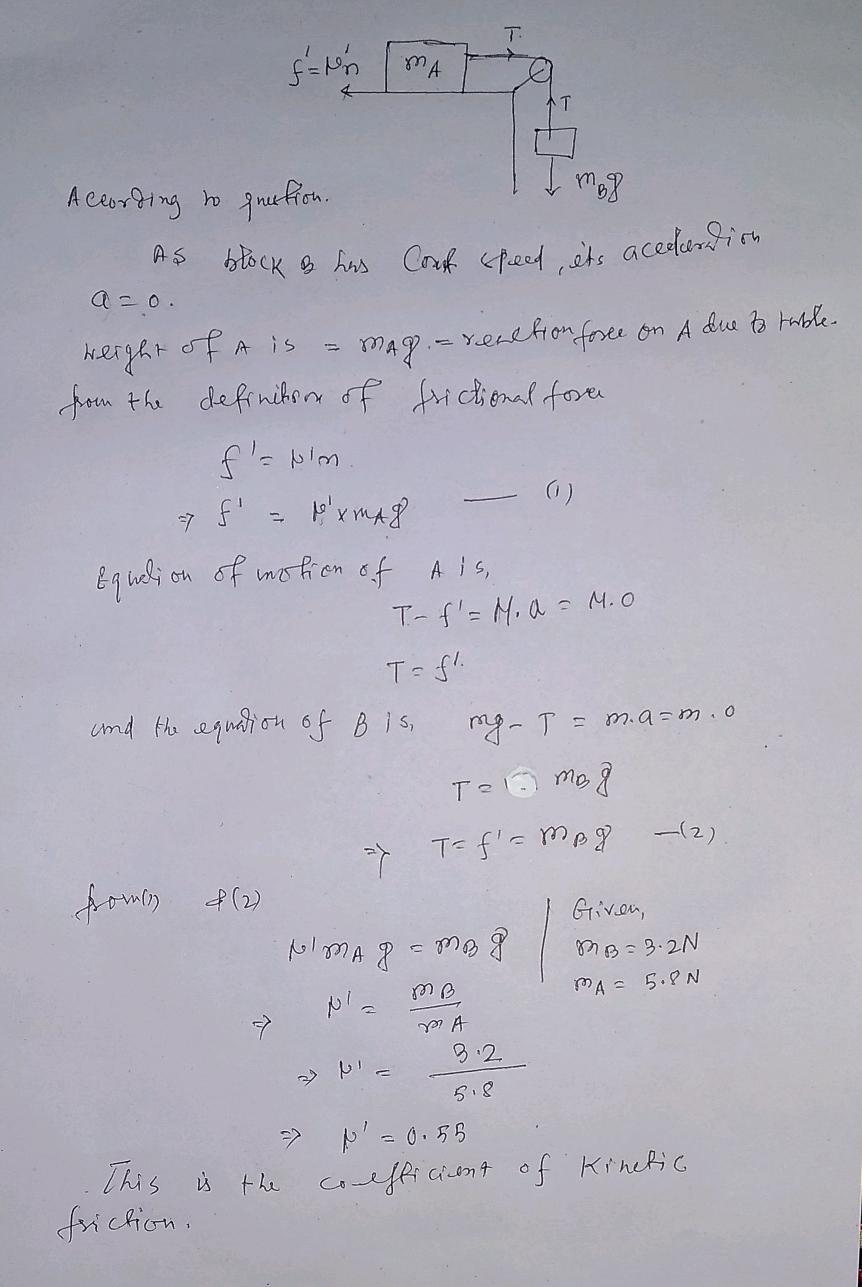#### Earn Coin

Coins can be redeemed for fabulous gifts.

Similar Homework Help Questions
• ### Consider the system shown in the figure.(Figure 1) Block A has weight 5.4 N and block...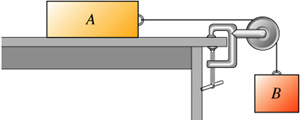Consider the system shown in the figure.(Figure 1) Block A has weight 5.4 N and block B has weight 3.6 N . Once block B is set into downward motion, it descends at a constant speed. Assume that the mass and friction of the pulley are negligible. Calculate the coefficient of kinetic friction μ between block A and the table top. Express your answer numerically. μ =

• ### ReviewI ConstantsI Periodic Table Part A Consider the system shown in the figure.(Figure 1) Block A...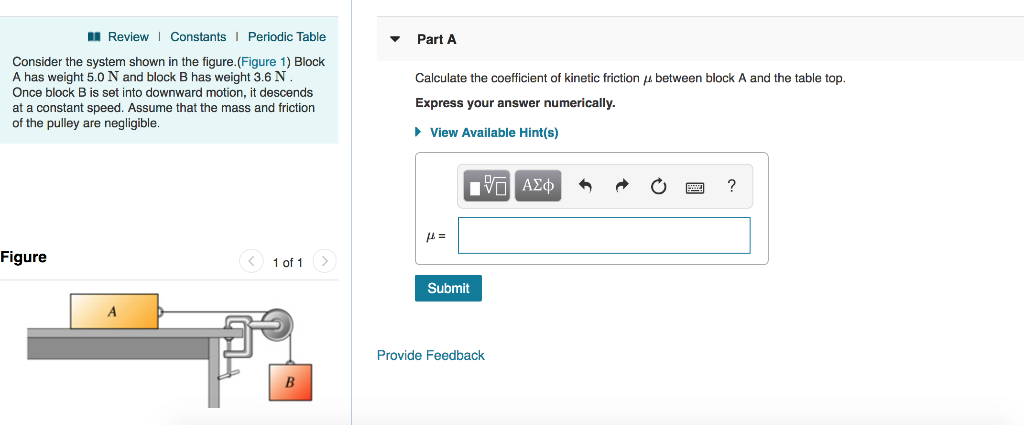ReviewI ConstantsI Periodic Table Part A Consider the system shown in the figure.(Figure 1) Block A has weight 5.0 N and block B has weight 3.6 N Once block B is set into downward motion, it descends at a constant speed. Assume that the mass and friction of the pulley are negligible Calculate the coefficient of kinetic friction between block A and the table top. Express your answer numerically View Available Hint(s) Figure 1 of 1 Submit Provide Feedback

• ### Consider the system shown in the figure.(Figure 1) Block A has weight 5.0 N and block...

Consider the system shown in the figure.(Figure 1) Block A has weight 5.0 N and block B has weight 3.0 N . Once block B is set into downward motion, it descends at a constant speed. Assume that the mass and friction of the pulley are negligible. Calculate the coefficient of kinetic friction μ between block A and the table top.

• ### Consider the system shown in the figure. Block A has weight 4.91 N and block B has weight 2.94 N. Once block B is set in...

Consider the system shown in the figure. Block A has weight 4.91 N and block B has weight 2.94 N. Once block B is set into downward motion, it descends at a constant speed. Assume that the mass and friction of the pulley are negligible. Calculate the coefficient of kinetic friction mu between block A and the table top. I'm not sure where to begin.

• ### Item 21 21 of 25 Consider the system shown in the figure.(Figure 1) Block A has...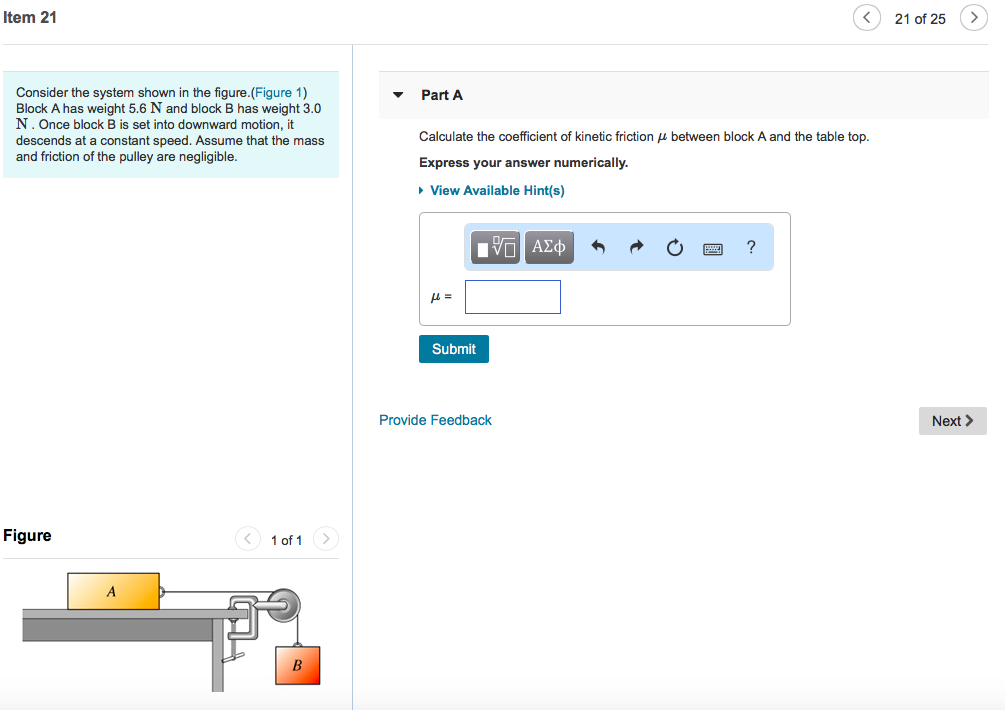Item 21 21 of 25 Consider the system shown in the figure.(Figure 1) Block A has weight 5.6 N and block B has weight 3.0 N. Once block B is set into downward motion, it descends at a constant speed. Assume that the mass Part A Calculate the coefficient of kinetic friction μ between block A and the table top. Express your answer numerically. y are negligible. View Available Hint(s) μ= Submit Provide Feedback Next > Figure 1 of 1

• ### Kinetic Friction in a block pulley systemConsider the system shown in the figure. Block A has weight4.91 and block B has weight2.94 .Once block B is set intodownward motion, it descends at a constant speed. Assume that themass and friction of the pulley are negligible. Calculate thecoefficient ofkinetic friction between block A and the table top.

• ### Consider the system shown in the figure below. Block A weighs 43.2 N and block B...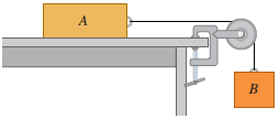Consider the system shown in the figure below. Block A weighs 43.2 N and block B weighs 29.0 N. Once block B is set into downward motion, it descends at a constant speed. (a) Calculate the coefficient of kinetic friction between block A and the tabletop. (b) A cat, also of weight 43.2 N, falls asleep on top of block A. If block B is now set into downward motion, what is its acceleration? magnitude m/s2 direction ---Select---upwarddownward Help!! Consider...

• ### mastering physics problem

Consider the system shown in the figure. Block A has weight 4.91 {rm N} and block B has weight 2.94 {rm N}. Once block B is set into downward motion, it descends ata constant speed. Assume that the mass and friction of the pulley are negligible.Calculate the coefficient of kinetic friction mu between block A and the table top.

• ### Calculate the coefficient of kinetic friction mu between block A and the table top.

Block A has weight w_A and block B has weight w_B. Once block B is set into downward motion, it descends at a constant speed. Assume that the mass and friction of thepulley are negligible.Calculate the coefficient of kinetic friction mu between block A and the table top.

• ### Constants Part A Consider the system shown in the figure. The rope and pulley have negligible...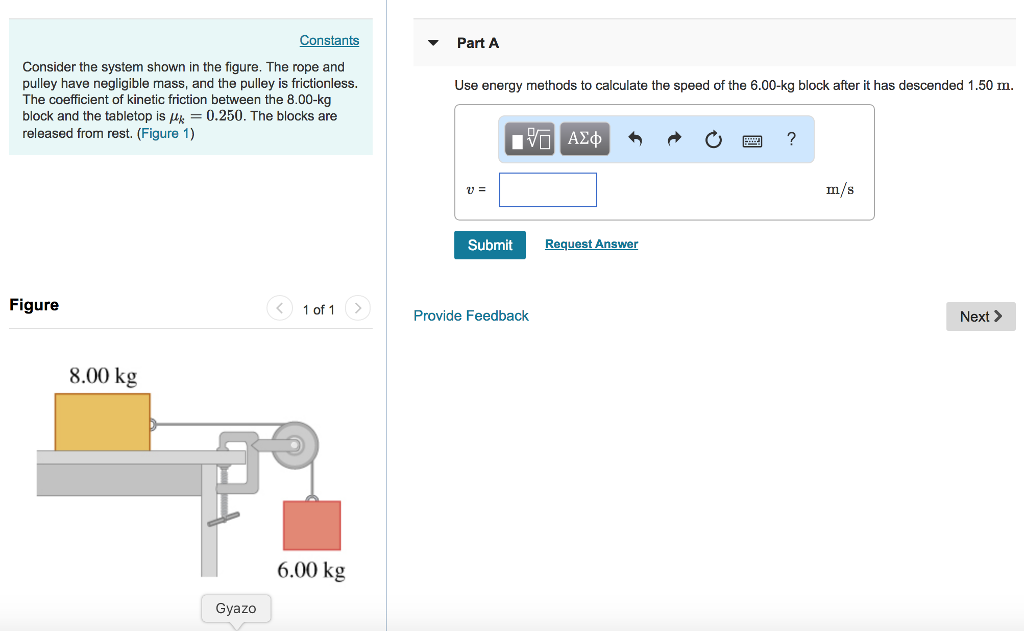Constants Part A Consider the system shown in the figure. The rope and pulley have negligible mass, and the pulley is frictionless. The coefficient of kinetic friction between the 8.00-kg block and the tabletop is0.250. The blocks are released from rest. (Figure 1) Use energy methods to calculate the speed of the 6.00-kg block after it has descended 1.50 m m/s Submit Request Answer Figure 1 of 1 Provide Feedback Next> 8.00 kg 6.00 kg Gyazo